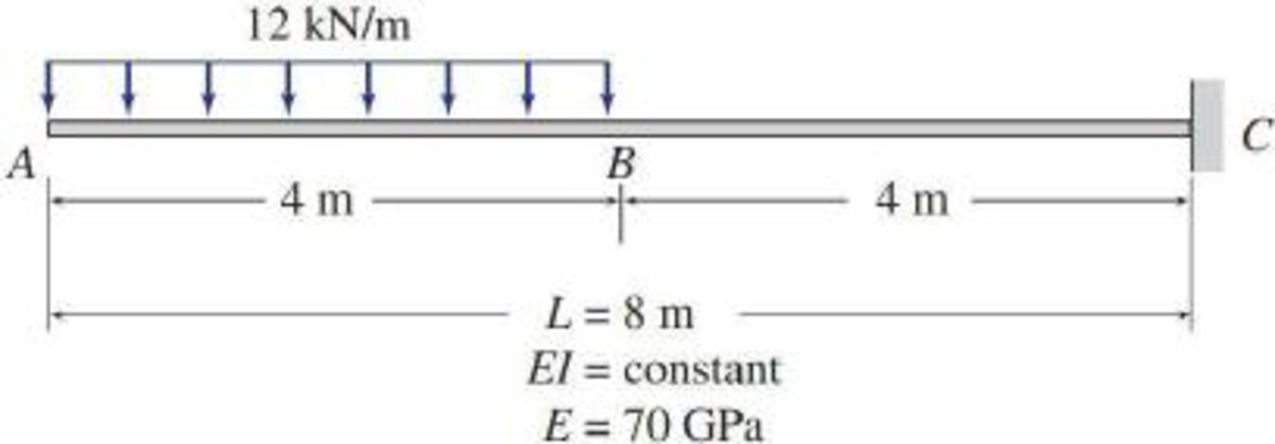# 6.44 through 6.48 Using the conjugate-beam method, determine the smallest moments of inertia I required for the beams shown in Figs. P6.18 through P6.22, so that the maximum beam deflection does not exceed the limit of 1/360 of the span length (i.e., Δ max ≤ L /360). FIG. P6.20, P6.46

#### Solutions

Chapter
Section
Chapter 6, Problem 46P
Textbook Problem
87 views

## 6.44 through 6.48 Using the conjugate-beam method, determine the smallest moments of inertia I required for the beams shown in Figs. P6.18 through P6.22, so that the maximum beam deflection does not exceed the limit of 1/360 of the span length (i.e., Δmax ≤ L/360).FIG. P6.20, P6.46To determine

Find the smallest moment of inertia (I) required for the beam if the deflection does not exceed the limit of l/360 of the span length by using conjugate beam method.

### Explanation of Solution

Given information:

The Young’s modulus (E) is 200 GPa.

Calculation:

Consider the flexural rigidity EI of the beam is constant.

Show the free body diagram of the given beam as in Figure (1).

Refer Figure (1),

Consider upward is positive and downward is negative.

Consider clockwise is negative and counterclockwise is positive.

Since support A is a free end there will be no reaction. Therefore, the reaction at support A is automatically zero.

Determine the support reaction at C using the Equation of equilibrium;

V=0RC(12×4)=0RC=48kN

Determine the moment at point C;

MC=(12×4×(42+4))=288kNm

Determine the moment at point B;

MB=(12×4×(42))=96kNm

Determine the moment at point A;

MA=48×8(12×4×(42))288=38496288=0

Show the M/EI diagram of the given beam as in Figure (2).

Conjugate-beam method:

In the given beam system, point C is a fixed end and point A is free end. But in the conjugate-beam method the fixed end of a real beam becomes free and the free end of real beam changed into the fixed end.

Show the M/EI diagram for the conjugate-beam as in Figure (3).

Consider the external forces acting (right of A) upward on the free body diagram as positive.

Determine the deflection at A using the relation;

ΔA=ΔAC=[13×b1×h1(34×b1)(b2×h2)(b1+b22)12×b3×h3(23×b3+b1)]

Here, b is the width and h is the height of respective parabola, rectangle, and triangle.

Substitute 4 m for b1, 96EI for h1, 4 m for b2, 96EI for h2, 4 m for b3, and (288EI96EI) for h3

### Still sussing out bartleby?

Check out a sample textbook solution.

See a sample solution

#### The Solution to Your Study Problems

Bartleby provides explanations to thousands of textbook problems written by our experts, many with advanced degrees!

Get Started

Find more solutions based on key concepts
For Problem 19.22, calculate the probability distribution and plot the probability distribution curve.

Engineering Fundamentals: An Introduction to Engineering (MindTap Course List)

Repeat Problem 2-1 without the possibility of rain accumulation on the roof.

Steel Design (Activate Learning with these NEW titles from Engineering!)

Define a class, subclass, and superclass, and provide three examples of each.

Systems Analysis and Design (Shelly Cashman Series) (MindTap Course List)

What is (are) the major alloying element(s) of 6061 aluminum?

Precision Machining Technology (MindTap Course List)

Find the resultant of the three forces acting on the square plate.

International Edition---engineering Mechanics: Statics, 4th Edition

List examples of desktop and mobile operating systems.

Enhanced Discovering Computers 2017 (Shelly Cashman Series) (MindTap Course List)

What is the first weld bead of a multiple pass weld called?

Welding: Principles and Applications (MindTap Course List)

If your motherboard supports ECC DDR3 memory, can you substitute non-ECC DDR3 memory?

A+ Guide to Hardware (Standalone Book) (MindTap Course List)